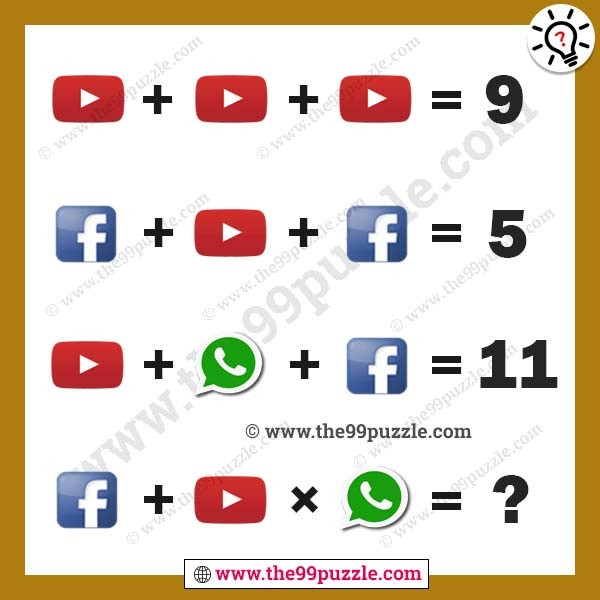# 13 Tricky puzzles that will totally blow your brain

In this post, you will find 13 tricky puzzles that will totally blow your brain. There are many different types of brain teasers that help to improve your logical thinking. Nowadays logical puzzles are asked in many examinations and interviews. If you are a genius you can answer all of these brain teaser puzzles.

1. Which Glass Has More Water with Answer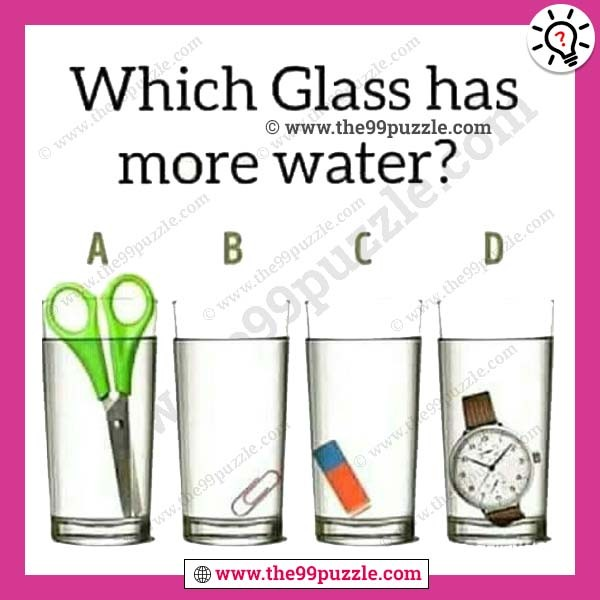2. What three numbers have the same answer when added together and multiplied together?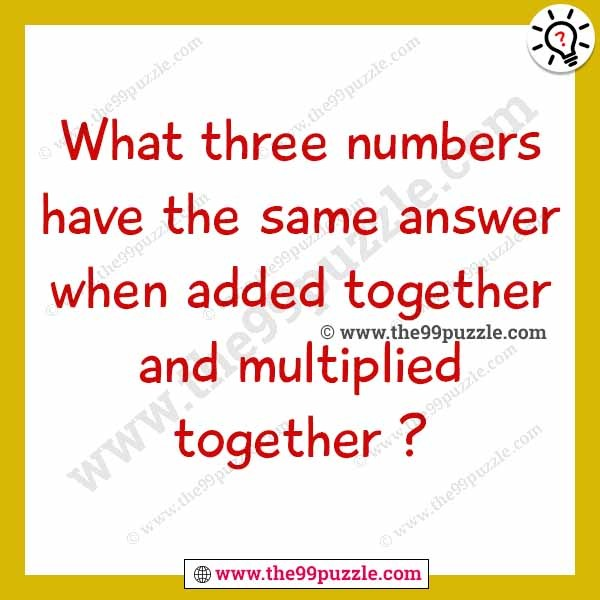3. Logical reasoning letter puzzles for adults

A+A=B, B+B=D, A+D=E, B+D+E=?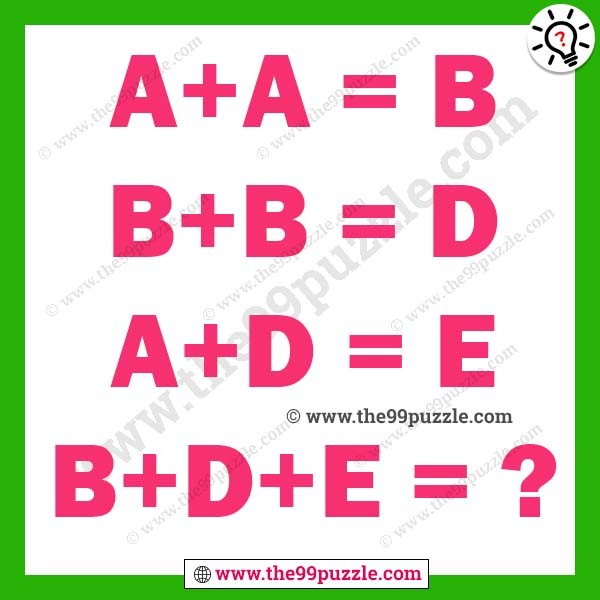4. Can you crack the lock code?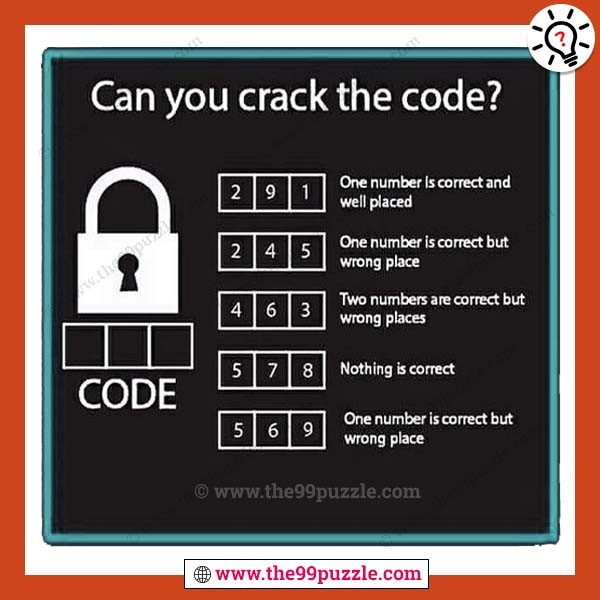5. Shoes Man Specs Boxing Glove Equation Puzzle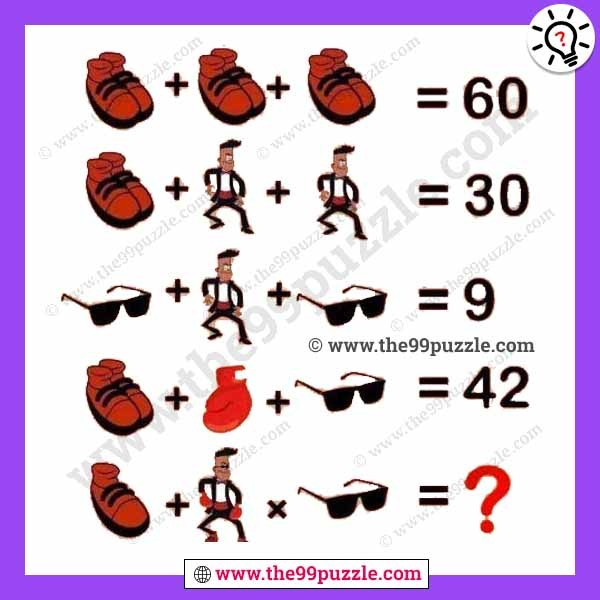6. Tricky letter puzzles for teens

1111=r, 2222=t, 3333=e, 4444=n, 5555=?7. Shoe man whistle picture math puzzle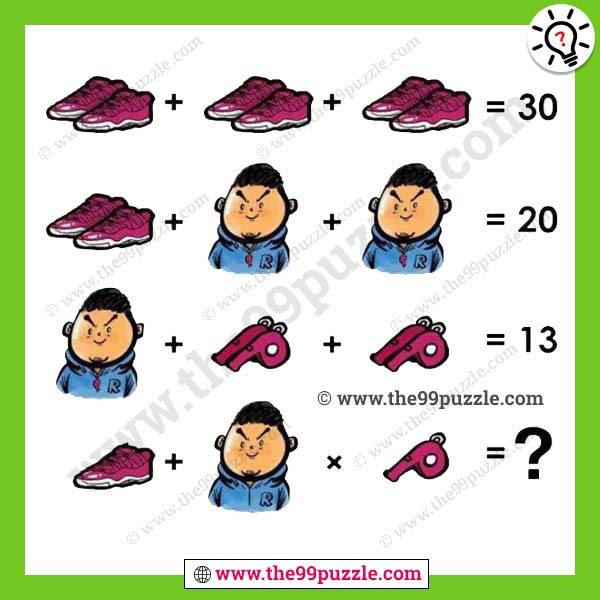8. Which letter replaces the question mark?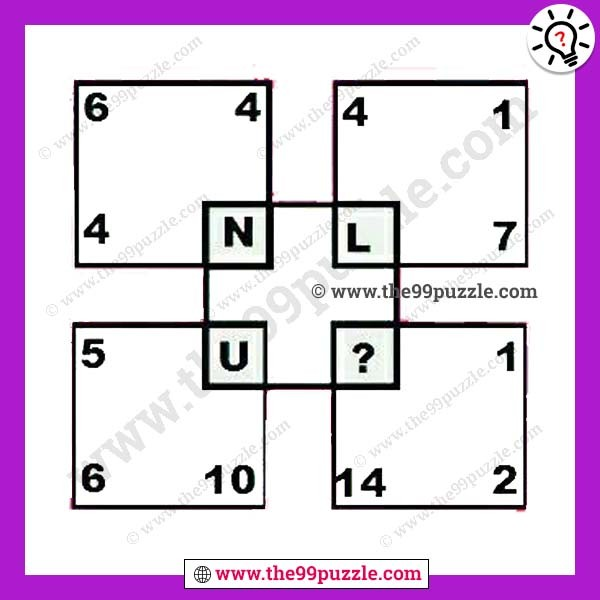9. Maths logical equation brain teaser with answer

1+1=6, 2+5=7, 3+4=9, 6+8=8, 7+9=?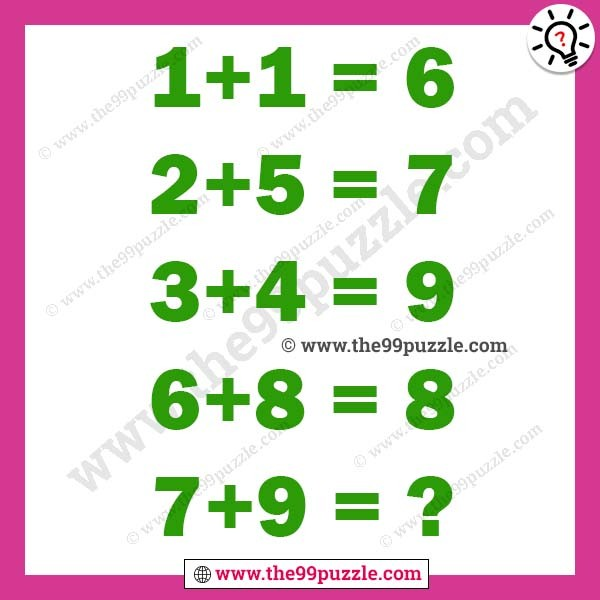10. What number can replaces the question mark?11. Can you identify the picture murder or suicide?12. Social media picture puzzles with answer